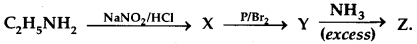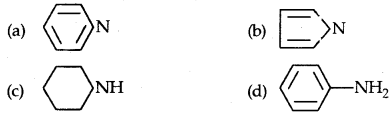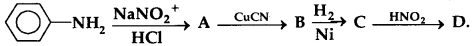# MCQ Questions for Class 12 Chemistry Chapter 13 Amines with Answers

We have compiled the NCERT MCQ Questions for Class 12 Chemistry Chapter 13 Amines with Answers Pdf free download covering the entire syllabus. Practice MCQ Questions for Class 12 Chemistry with Answers on a daily basis and score well in exams. Refer to the Amines Class 12 MCQs Questions with Answers here along with a detailed explanation.

## Amines Class 12 MCQs Questions with Answers

Question 1.
Which of the following does not react with Hinsberg reagent?
(a) Ethylamine
(b) (CH3)2NH
(c) (CH3)3N
(d) Propan-2-amine

Question 2.above sequence, Z is
(a) Cyanoethane
(b) Ethanamide
(c) Methanamine
(d) Ethanamine

Question 3.
Oxidation of aniline with K2Cr2O7/H2SO4 gives
(a) phenylhydroxylamine
(b) p-benzoquinone
(c) nitrosobenzene
(d) nitrobenzene

Question 4.
Which of the following amines can exhibit enantiomerism?
(a) Benzeamine
(b) 2-Butanamine
(c) 2-Propanamine
(d) 2-Methyl-propanamine.

Question 5.
Which of the following: when heated with a mixture of ethanmine and alcoholic potash gives ethyl isocyanide?
(a) 2-chloropropane
(b) 2,2-dichloropropane
(c) trichloromethane
(d) tetrachloromethane

Question 6.
Which of the following pair of species will yield carbylamine?
(a) CH3CH2Br and KCN
(b) CH3CH2Br and NH3 (excess)
(c) CH3CH2Br and AgCN
(d) CH3CH2NH2 and HCHO

Question 7.
Which one of the following methods is neither meant for the synthesis nor for separation of amines?
(a) Hinsberg method
(b) Hoffmann method
(c) Wurtz reaction
(d) Curticus reaction

Question 8.
C6H5CONHCH3 can be converted into C6H5CH2NHCH3 by
(a) NaBH4
(b) H2-Pd/C
(c) LiAlH4
(d) Zn-Hg/HCl

Question 9.
C6H5N+2 Cl + CuCN → C6H5CN + N2 + CuCl. The above chemical reaction is associated with which of the following name:
(a) Balz Schiemen
(b) Gattermann
(c) Shimonini
(d) Sandmeyer.

Question 10.
The reaction of aniline with benzoyl chloride gives
(a) Benzoin
(b) Benzanilide
(c) Benzalaniline
(d) Benzamide

Question 11.
Aniline was diazotised and is subsequently reduced with tin (II) chloride and HCl. The product formed is
(a) Phenylhydrazine
(b) Aniline
(c) Phenyl aniline
(d) p-aminoazobenzene.

Question 12.
Which of the following will not give primary amine
(a) Dehydration of amide
(b) Acidic hydrolysis of alkyl isocyanides
(c) Reduction of amides
(d) Reduction of alkyl cyanides.

Question 13.
The reagents used in Hoffman’s Mustard oil reaction are
(a) Mustred oil and 1° amine
(b) CS2 and aniline in HgCl2
(c) Nitrobenzene and CS2
(d) Sand RNC.

Answer: (b) CS2 and aniline in HgCl2

Question 14.
Which of the following cannot couple with benzene diazonium chloride?
(a) Aniline
(b) Phenol
(c) ß-Napthol
(d) Benzyl alcohol.

Question 15.
The hybrid state of N is R2NH is
(a) sp³
(b) sp²
(c) sp
(d) dsp²

Question 16.
The strongest base among the following is?Question 17.
Which of the following is the strongest base?Question 18.
Among the following, weakest base is
(a) C6H5CH NH2
(b) C6H5CH2NHCH3
(c) O2N-CH2NH2
(d) CH3CONH2

Question 19.
Among the following, the dissociation constant is highest for
(a) C6H5OH
(b) C6H5CH2OH
(c) CH3-C = CH
(d) CH3N+H3Cl

Question 20.
Intermolecular hydrogen bonding is strongest in
(a) Methylamine
(b) Phenol
(c) Formaldehyde
(d) Methanol

Question 21.
Reaction of cyclohexanone with dimethylamine in the presence of catalytic amount of an acid forms a compound if water formed during the reaction is continuously removed. The compound formed is generally known as
(a) an enamine
(b) a Schiff’s base
(c) an amine
(d) an imine.

Question 22.
Among the following the most basic compound is
(a) benzylamine
(b) aniline
(c) acetanilide
(d) p-nitroaniline

Question 23.
Aniline in a set of reactions yielded a product D.The structure of D would be
(a) C6H5CH2OH
(b) C6H5CH2NH2
(c) C6H5NHOH
(d) C6H5NHCH2CH3.

Question 24.
Reaction of aniline with benzaldehyde is
(a) Substitution
(c) Condensation
(d) polymerisation

Question 25.
Which is more basic?
(a) Benzylamine
(b) aniline
(c) Acetamide
(d) None of these

Question 26.
The compound that will react most readily with NaOH to form methanol is
(a) (CH3)4N+I
(b) CH3OCH3
(c) (CH3)3SI
(d) (CH3)3CCl

Question 27.
The correct order of increasing basic nature for the bases NH3, CH3NH2 and (CH3)2 NH is
(a) CH3 NH2 < (CH3)2 NH < NH3
(b) CH3NH2 < NH3 < (CH3)2NH
(c) (CH3)2 NH< NH3 < CH3NH2
(d) NH3 < CH3NH2 < (CH3)2NH

Answer: (d) NH3 < CH3NH2 < (CH3)2NH

Question 28.
Aniline first reacts with acetyl chloride producing compound A. A reacts with $$\frac {HNO_3}{H_2SO_4}$$ mixture and produces comp and B which hydrolyses to compound C. What is the identity of C?
(a) Acetanilide
(b) p-Nitroacetanilide
(c) p-Nitroaniline
(d) Sulphanilic acid.

Question 29.
Which is most basic?
(a) C6H5NH2
(b) (C6H5)2NH
(c) CH3NH2
(d) (CH3)2NH

Question 30.
The amine that does not react with acetyl chloride is
(a) CH3NH2
(b) (CH3)2NH
(c) (CH3)3 N
(d) none of these